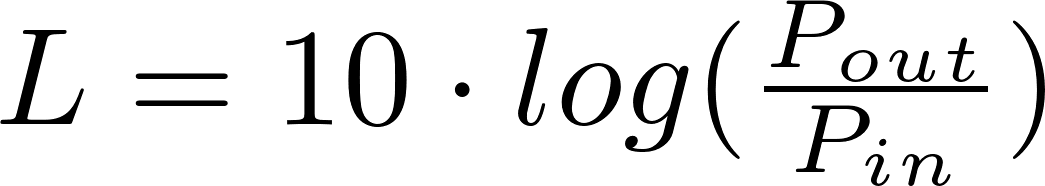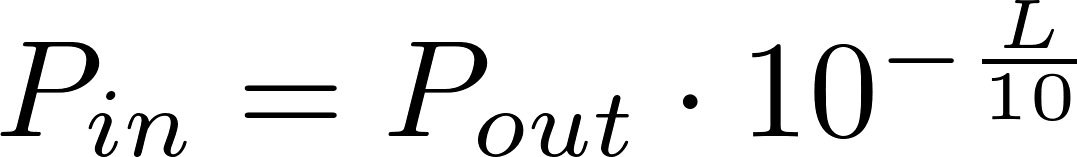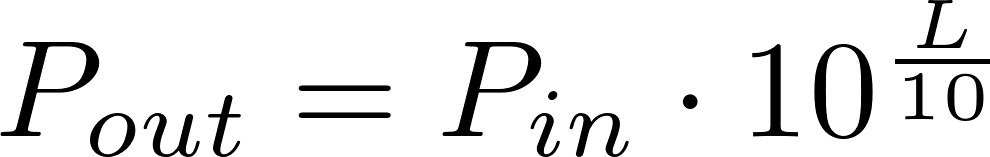# Decibel (power)

Electric power is the rate at which energy is transferred by a circuit. The symbol for power is P and it is measured in watt (W). The Watt is defined as one joule per second. Electric power is equal to the voltage multiplied by the current.

### Formulas

The following formula can be used to calculate the decibel value for two electric powers or one of the powers.P in is the symbol for the input power and is measured in watt (W).
P out is the symbol for the output power and is measured in watt (W).
L is the symbol for the decibel value and is measured in dB.